61 Posts
1 , 2 , 3  Next »   |

Subject: Virtuacon - "An Above Average Night in the Woods" contestYour Tags: Add tags Popular Tags: [View All]
George Buss
United States
Muncie
Indiana
"It seems, that is, I may have become, quite by accident, the chosen, what what?"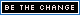“Here is my secret. It's quite simple: One sees clearly only with the heart. Anything essential is invisible to the eyes.” ― Antoine de Saint-Exupéry, Petit PrinceThis is an unofficial contest for Virtuacon. I've enjoyed the games, panels and contests I've participated in so much, that I wanted to add to the fun.

----------

You and some of your friends from RPGGeek decide to take a break from all this online gaming to play your favorite RPG out in the woods. It took a bit of a hike, but the campsite is set. Large tent, stash of food, and a ready supply of the beverage of your choice makes it the perfect night. The full moon is just icing.

All is going well. You are about two thirds through your home brew when a nearby sound sends chills up your spine. The look on your friends faces tells you the worst. They heard it too. As if one man, you all turn your heads at the same time like a slightly overweight synchronized swimming team out of water. There is a sound and you smell urine. Someone just wet themselves. It might have been you.

Half crouched at the edge of the clearing is a man, or some grotesque imitation. It's body is covered in a scraggly fur coat, matted and glistening at parts. When the thing turns its head, you realize the worst. The pointed ears and long rounded muzzle revealed by silhouette in the moonlight can mean only one thing: werewolf.The creature stands to its full height. It arches it's back and lifts its chin to the Hunter's Moon to call down its blessing with a howl. None of you see what it does next; you've all turned tail and started running.

Your life flashed before your eyes as you run, stopping suddenly on a memory of High School Gym Class. Coach is running beside you. He's screaming in your ear.

"Hey, Punk! You gonna be a loser today? Listen to me! You don't need to be! You just gotta stay ahead of the guy behind you. You only gotta beat one! Be faster than the other guy. For Pete's Sake, for once in your life, you gotta be above average!"

The Ghost of Coach disappears with a snarl as one of your buddies behind you falls to the were-creatures claws, but his words ring in your ears, "You gotta be above average!" The irony is not lost on you. Coach may just save your life.

----------

Time to Run!

Post a comment below with a link to one RPG that you feel is above average.

Now, Run!

To run, you need to add a geek roll 1 die. You need to roll higher than the average roll with those dice. Choose the die type you wish to use and stick with that type (d4, d6, d8, d10, d12, d20, or d100). Do not change it up.

Then start by rolling 1 die. If you roll above the average, edit your comment and roll again, adding one more die of your chosen type. Stop when you roll below average. So your comment will look something like this:

RUN! 1d20 = (19) = 19
RUN AGAIN! 1d20 + 1d20 = (17) + (1) = 18
RUN RUN RUN!!! 1d20 + 1d20 + 1d20 = (10) + (9) + (17) = 36

You can stop at anytime while you are still above average. The player who gets the farthest while staying above average will be the winner. Ties will be determined by the status of the last roll: above or below average.

----------
The formula to determine the average is X(Y+1)/2 where X equals # of Dice and Y equals sides on the Die. So a the average of a 3d6 would be 3(6+1)/2 or 10.5. To be higher than average, you would need to roll a total of 11 with 3d6. Average of 3d20 would be 31.5 so you need to roll a 32 or better.
----------

Prizes:
First place will not be eaten by a werewolf.
The rest of you may not make it.
(I'm not sure what the prize will be, but press your luck all the same!)

Thanks again to all the folks who have made Virtuacon awesome!

NOW, RUN!!!

• [+] Dice rolls
• [+]
• 449759. ThatFedoraGuy
• 1d20 =
• (19) =
• 19
• RUN!
• Sun Oct 20, 2013 12:15 am
• [+]
• 449760. ThatFedoraGuy
• 1d20 + 1d20 =
• (17) + (1) =
• 18
• RUN AGAIN!
• Sun Oct 20, 2013 12:15 am
• [+]
• 449761. ThatFedoraGuy
• 1d20 + 1d20 + 1d20 =
• (10) + (9) + (17) =
• 36
• RUN RUN RUN!!!
• Sun Oct 20, 2013 12:15 am
George Buss
United States
Muncie
Indiana
"It seems, that is, I may have become, quite by accident, the chosen, what what?"“Here is my secret. It's quite simple: One sees clearly only with the heart. Anything essential is invisible to the eyes.” ― Antoine de Saint-Exupéry, Petit Prince
Mouse Guard Roleplaying Game

RUN! 1d20 = (8) = 8

AAAAUUUUUGGGGGhhhhhhh....burbblblblblbllllll...
• [+] Dice rolls
• [+]
• 449765. ThatFedoraGuy
• 1d20 =
• (8) =
• 8
• RUN!
• Sun Oct 20, 2013 12:26 am
Monsterhearts

Now RUN!
1d12 = (4) = 4
Aaaaargh! (wilhelm scream)
• [+] Dice rolls
• [+]
• 449766. Conlaen
• 1d12 =
• (4) =
• 4
• Sun Oct 20, 2013 12:31 am
My Above Average RPG: Pathfinder Roleplaying Game (1st Edition)

RUN 1d4 = (3) = 3
RUN RUN 1d4+1d4 = (4) + (4) = 8
RUN RUN AND DONE! 1d4+1d4+1d4 = (2) + (1) + (3) = 6

Aaaaaaarrrrrggghhh!• [+] Dice rolls
• [+]
• 449767. wavemotion
• 1d4 =
• (3) =
• 3
• RUN
• Sun Oct 20, 2013 12:31 am
• [+]
• 449770. wavemotion
• 1d4+1d4 =
• (4) + (4) =
• 8
• RUN RUN
• Sun Oct 20, 2013 12:33 am
• [+]
• 449772. wavemotion
• 1d4+1d4+1d4 =
• (2) + (1) + (3) =
• 6
• RUN RUN AND DONE!
• Sun Oct 20, 2013 12:34 am
later! 1d20 = (17) = 17
Zoom! 1d20 + 1d20 = (11) + (2) = 13
• [+] Dice rolls
• [+]
• 449769. Mulligans
• 1d20 =
• (17) =
• 17
• later!
• Sun Oct 20, 2013 12:32 am
• [+]
• 449771. Mulligans
• 1d20 + 1d20 =
• (11) + (2) =
• 13
• Zoom!
• Sun Oct 20, 2013 12:34 am
This would be easier if I wasn't wearing boots. Guess its my Fate.

1d20 = (16) = 16
2d20 = (13 + 17) = 30
1d20+1d20+1d20 = (7) + (15) + (12) = 34
And I stop here to look back upon my mangled friends who were so kind as to run slower than me. Hopefully none of them pass me up as I do so.
...
And then I re-read the rules posted on the sign behind the werewolf and turn to run some more!
1d20 + 1d20 + 1d20 + 1d20 = (8) + (10) + (11) + (6) = 35
• [+] Dice rolls
• [+]
• 449773. cornjerker
• 1d20 =
• (16) =
• 16
• Sun Oct 20, 2013 12:35 am
• [+]
• 449776. cornjerker
• 2d20 =
• (13 + 17) =
• 30
• Sun Oct 20, 2013 12:38 am
• [+]
• 449778. cornjerker
• 1d20+1d20+1d20 =
• (7) + (15) + (12) =
• 34
• Sun Oct 20, 2013 12:41 am
• [+]
• 449791. cornjerker
• 1d20 + 1d20 + 1d20 + 1d20 =
• (8) + (10) + (11) + (6) =
• 35
• Sun Oct 20, 2013 12:53 am
This is turning out to be a Fiasco

It 8 my friend! 1d8 = (4) = 4
• [+] Dice rolls
• [+]
• 449777. nyriv
• 1d8 =
• (4) =
• 4
• It 8 my friend!
• Sun Oct 20, 2013 12:40 am
InSpectres

RUN! 1d6 = (5) = 5
RUN AGAIN! 1d6 + 1d6 = (6) + (6) = 12
RUN MORE! 1d6 + 1d6 + 1d6 = (3) + (3) + (5) = 11
RUN RUN! 1d6 + 1d6 + 1d6 + 1d6 = (6) + (6) + (5) + (4) = 21
RUUUUNNNN! 1d6 + 1d6 + 1d6 + 1d6 + 1d6 = (1) + (1) + (3) + (2) + (4) = 11 Crud!
• [+] Dice rolls
• [+]
• 449779. fuzzydice82
• 1d6 =
• (5) =
• 5
• RUN!
• Sun Oct 20, 2013 12:42 am
• [+]
• 449780. fuzzydice82
• 1d6 + 1d6 =
• (6) + (6) =
• 12
• RUN AGAIN!
• Sun Oct 20, 2013 12:43 am
• [+]
• 449781. fuzzydice82
• 1d6 + 1d6 + 1d6 =
• (3) + (3) + (5) =
• 11
• RUN MORE!
• Sun Oct 20, 2013 12:43 am
• [+]
• 449782. fuzzydice82
• 1d6 + 1d6 + 1d6 + 1d6 =
• (6) + (6) + (5) + (4) =
• 21
• RUN RUN!
• Sun Oct 20, 2013 12:46 am
• [+]
• 449784. fuzzydice82
• 1d6 + 1d6 + 1d6 + 1d6 + 1d6 =
• (1) + (1) + (3) + (2) + (4) =
• 11
• RUUUUNNNN!
• Sun Oct 20, 2013 12:46 am
Mini Six is my poison of choice.

Rolling my 4d6 Athletics skill...

Gangway! 4d6 = (3 + 2 + 4 + 4) = 13

Chomp, crunch, crunch, chomp.
• [+] Dice rolls
• [+]
• 449783. MonsterMike
• 4d6 =
• (3 + 2 + 4 + 4) =
• 13
• Sun Oct 20, 2013 12:46 am
Gotta be Advanced Dungeons & Dragons (1st Edition)

Run! 1d12 = (11) = 11
Run!! 2d12 = (11 + 6) = 17
RUN! 3d12 = (8 + 10 + 2) = 20
RUN!! 4d12 = (8 + 5 + 10 + 8) = 31
Run! 5d12 = (11 + 6 + 4 + 5 + 8) = 34
Run!! 6d12 = (2 + 2 + 3 + 1 + 7 + 6) = 21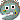Ouch• [+] Dice rolls
• [+]
• 449815. Roplesaurus
• 1d12 =
• (11) =
• 11
• Sun Oct 20, 2013 1:34 am
• [+]
• 449816. Roplesaurus
• 2d12 =
• (11 + 6) =
• 17
• Sun Oct 20, 2013 1:35 am
• [+]
• 449818. Roplesaurus
• 3d12 =
• (8 + 10 + 2) =
• 20
• Sun Oct 20, 2013 1:35 am
• [+]
• 449819. Roplesaurus
• 4d12 =
• (8 + 5 + 10 + 8) =
• 31
• Sun Oct 20, 2013 1:36 am
• [+]
• 449821. Roplesaurus
• 5d12 =
• (11 + 6 + 4 + 5 + 8) =
• 34
• Sun Oct 20, 2013 1:37 am
• [+]
• 449824. Roplesaurus
• 6d12 =
• (2 + 2 + 3 + 1 + 7 + 6) =
• 21
• Sun Oct 20, 2013 1:38 am
I'll go with Dread...

d100 1d100 = (89) = 89
Still going... 2d100 = (38 + 71) = 109
That was close... 3d100 = (72 + 4 + 88) = 164
Cutting it pretty fine... 4d100 = (33 + 81 + 74 + 66) = 254
Keep running... 5d100 = (69 + 96 + 9 + 54 + 76) = 304
Still going... 6d100 = (16 + 80 + 97 + 27 + 54 + 5) = 279

Argh!!
• [+] Dice rolls
• [+]
• 449827. E Decker
• 1d100 =
• (89) =
• 89
• d100
• Sun Oct 20, 2013 1:41 am
• [+]
• 449828. E Decker
• 2d100 =
• (38 + 71) =
• 109
• Still going...
• Sun Oct 20, 2013 1:43 am
• [+]
• 449829. E Decker
• 3d100 =
• (72 + 4 + 88) =
• 164
• That was close...
• Sun Oct 20, 2013 1:44 am
• [+]
• 449830. E Decker
• 4d100 =
• (33 + 81 + 74 + 66) =
• 254
• Cutting it pretty fine...
• Sun Oct 20, 2013 1:45 am
• [+]
• 449831. E Decker
• 5d100 =
• (69 + 96 + 9 + 54 + 76) =
• 304
• Keep running...
• Sun Oct 20, 2013 1:46 am
• [+]
• 449832. E Decker
• 6d100 =
• (16 + 80 + 97 + 27 + 54 + 5) =
• 279
• Still going...
• Sun Oct 20, 2013 1:47 am
I'm running The Quiet Year in a couple of hours...

How many weeks will this project take? 1d6 = (5) = 5
Not sure I can run that long without stopping. Grab some berries off the bushes as I run past 2d6 = (3 + 3) = 6

Ack, poisonous berries! The stomach cramps slow me down just enough to... AAAAUUUGGGGGHHHH
• [+] Dice rolls
• [+]
• 449834. baychang
• 1d6 =
• (5) =
• 5
• How many weeks will this project take?
• Sun Oct 20, 2013 2:14 am
• [+]
• 449837. baychang
• 2d6 =
• (3 + 3) =
• 6
• Not sure I can run that long without stopping. Grab some berries off the bushes as I run past
• Sun Oct 20, 2013 2:17 am
Traveller (Classic)

Run 1d100 = (50) = 50

Talk about cutting it close and losing. Now that I'm infected by lycanthropy, what neat bonuses do I get? Oh, I'm werewolf chow, that's not so much aaaarrrrgggghhhh!!!!

• [+] Dice rolls
• [+]
• 449839. paulidale
• 1d100 =
• (50) =
• 50
• Run
• Sun Oct 20, 2013 2:23 am
Okay, I'm going with my own: Dwarf Star
1d12 = (7) = 7
1d12 + 1d12 = (1) + (8) = 9
• [+] Dice rolls
• [+]
• 449840. XendoBreckett
• 1d12 =
• (7) =
• 7
• Sun Oct 20, 2013 2:28 am
• [+]
• 449841. XendoBreckett
• 1d12 + 1d12 =
• (1) + (8) =
• 9
• Sun Oct 20, 2013 2:28 am
Apologies for the interruption:
XendoBreckett wrote:
An Above Average Night Contest badge...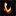Overtext: Virtuacon Contest: An Above Average Night - Don't press your luck!

(Hoping for some support so I can get a rush on this thing, as VC is already happening.)
Poll?
 Looks like a werewolf would be proud of the likeness. Looks like a werewolf ought to drag it through a field and devour it.
Poll created by XendoBreckett

Carry on.
• [+] Dice rolls
I'll go with Star Wars: Edge of the Empire

Run! 1d10 = (10) = 10
Run some more! 2d10 = (6 + 3) = 9

Hmmm... that certainly ain't no Kessel run.
• [+] Dice rolls
• [+]
• 449846. leemc13
• 1d10 =
• (10) =
• 10
• Sun Oct 20, 2013 3:07 am
• [+]
• 449847. leemc13
• 2d10 =
• (6 + 3) =
• 9
• Sun Oct 20, 2013 3:08 am
I'm going with "microscope"

RUN! 1d2 = (2) = 2
RUN!! 2d2 = (1 + 1) = 2
YUM!
• [+] Dice rolls
• [+]
• 449848. Dutys_Fist
• 1d2 =
• (2) =
• 2
• RUN!
• Sun Oct 20, 2013 3:10 am
• [+]
• 449849. Dutys_Fist
• 2d2 =
• (1 + 1) =
• 2
• RUN!!
• Sun Oct 20, 2013 3:10 am
Hopefully I can run as fast as Lady Blackbird!

RUNNING 1d12 = (9) = 9
FASTER! 1d12 + 1d12 = (5) + (7) = 12

OMNOMNOM!
• [+] Dice rolls
• [+]
• 449858. MichMedia
• 1d12 =
• (9) =
• 9
• RUNNING
• Sun Oct 20, 2013 5:17 am
• [+]
• 449859. MichMedia
• 1d12 + 1d12 =
• (5) + (7) =
• 12
• FASTER!
• Sun Oct 20, 2013 5:18 am
TMNT

Run 1d12 = (1) = 1

I turn and run straight into a tree before being eaten.
• [+] Dice rolls
• [+]
• 449860. bulldog93
• 1d12 =
• (1) =
• 1
• Run
• Sun Oct 20, 2013 5:30 am
I'll need warp 9 now as in Star Trek: The Role Playing Game

Run! 1d20 = (2) = 2

Crap. Warp core breach.
• [+] Dice rolls
• [+]
• 449876. Narl
• 1d20 =
• (2) =
• 2
• Run!
• Sun Oct 20, 2013 7:16 am
Earthdawn (1st Edition)

Run 1d12 = (10) = 10
Run! 2d12 = (2 + 10) = 12
• [+] Dice rolls
• [+]
• 449879. fastfox
• 1d12 =
• (10) =
• 10
• Run
• Sun Oct 20, 2013 7:31 am
• [+]
• 449880. fastfox
• 2d12 =
• (2 + 10) =
• 12
• Run!
• Sun Oct 20, 2013 7:31 am
George Buss
United States
Muncie
Indiana
"It seems, that is, I may have become, quite by accident, the chosen, what what?"“Here is my secret. It's quite simple: One sees clearly only with the heart. Anything essential is invisible to the eyes.” ― Antoine de Saint-Exupéry, Petit Prince
In a fitting irony, I spent the night playing a Pathfinder game with a Werewolf... And tonight's a full moon.

Awwwwiooooo!!!

Keep running guys!
• [+] Dice rolls
Jonna
Finland
Helsinki
Uusimaa
"Live your truth. Express your love. Share your enthusiasm. Take action towards your dreams. Walk your talk. Dance and sing to your music. Embrace your blessings. Make today worth remembering." ― Steve Maraboliनमस्ते (Namaste) – I honor the place in you in which the entire universe dwells. I honor the place in you which is of love, of truth, of light and of peace. When you are in that place in you and I am in that place in me, we are one.
Monster of the Week

Run! 1d10 = (2) = 2

Or don't run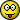• [+] Dice rolls
• [+]
• 449907. Kattvippa
• 1d10 =
• (2) =
• 2
• Run!
• Sun Oct 20, 2013 10:14 am
It Came from the Late, Late, Late Show (1st, 2nd & 3rd edition)

Run: 1d12 = (10) = 10
Run; 2d12 = (3 + 2) = 5

Argh.
• [+] Dice rolls
• [+]
• 449908. Mixula
• 1d12 =
• (10) =
• 10
• Sun Oct 20, 2013 10:45 am
• [+]
• 449909. Mixula
• 1d12 =
• (7) =
• 7
• Sun Oct 20, 2013 10:47 am
• [+]
• 449910. Mixula
• 1d12 =
• (11) =
• 11
• Sun Oct 20, 2013 10:50 am
• [+]
• 449911. Mixula
• 1d12 =
• (8) =
• 8
• Sun Oct 20, 2013 10:51 am
• [+]
• 449913. Mixula
• 2d12 =
• (3 + 2) =
• 5
• Sun Oct 20, 2013 10:54 am
Lasers & Feelings: I really feel I should run fast as a laser.

Run! (1d6) 1d6 = (4) = 4
Ruun! (2d6) 2d6 = (2 + 1) = 3

Well, it looks like I'm joining the ranks of those who unwillingly save somebody else's butt.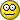• [+] Dice rolls
• [+]
• 449918. jasri
• 1d6 =
• (4) =
• 4
• Run! (1d6)
• Sun Oct 20, 2013 11:10 am
• [+]
• 449919. jasri
• 2d6 =
• (2 + 1) =
• 3
• Ruun! (2d6)
• Sun Oct 20, 2013 11:11 am
1 , 2 , 3  Next »   |